Next: ROBUST MODELING Up: Data modeling by least Previous: HOUSEHOLDER TRANSFORMATIONS AND GOLUB'S

# CHOICE OF A MODEL NORM

In recent years, a popular view of geophysical data modeling has been that the earth is a continuum and that we should regard the number of unknowns as infinite but the number of our observations as finite. In a computer one therefore approximates the situation by a highly underdetermined system of simultaneous equations. In order to get a unique answer, the solution should extremalize some integral. In practice, a sum of squares is often minimized in such a way as to produce a smooth solution. A typical mathematical formulation is to do a least squares fitting of an equation set like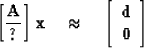(61)

where the top of block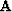denotes the underdetermined constraint equations with the data vector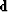;the unknowns are in the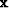vector; and the bottom block is a band matrix (all the nonzero elements cluster about the diagonal) which says that some filtered version ofshould vanish. The filter is often a roughing filter like the first-difference operator. In the absence of data, the first-difference operator leads to a constant solution which is sensible. What is not sensible is that it forces the result to be smoothed even though realistic earth models often contain step discontinuities (where two homogeneous media lie in contact).

The choice of a filter is a rather subjective matter, the choice often being made on the basis of the solution it will produce. Unfortunately, the solution is often a rather sensitive function of the subjectively chosen weights and filters; this fact makes the whole business an art, a matter of experience and judgment. General purpose theories of inversion exist, but they do not prepare the geophysicist to exploit the peculiarities of any particular situation or data set. Inversion theories, like mathematical statistics, should be used like a lamp post - to light the way, not to lean upon.

One useful concept in inversion theory is the idea of coordinate-system invariance. The idea is that one should get the same answer in an electrical conductivity problem whether one parameterizes the earth by an unknown conductivity at every point on a sufficiently dense mesh, or one parameterizes with resistivity on the mesh, or one parameterizes by coefficients of some expansion in a complete set of basis functions. Clearly, the idea of fitting low-order coefficients in some expansion setting the high-order coefficients equal to zero is not a coordinate-invariant approach. A different origin for polynomial expansions can change everything. A different set of basis functions would change everything. Of course, it is not essential to use a coordinate-system invariant technique in data inversion. But if one does not, one should beware of the sensitivity of one's solution to changes in the coordinate system.

 6-2 Figure 1 Minimizing either of these two functions will drive p toward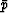.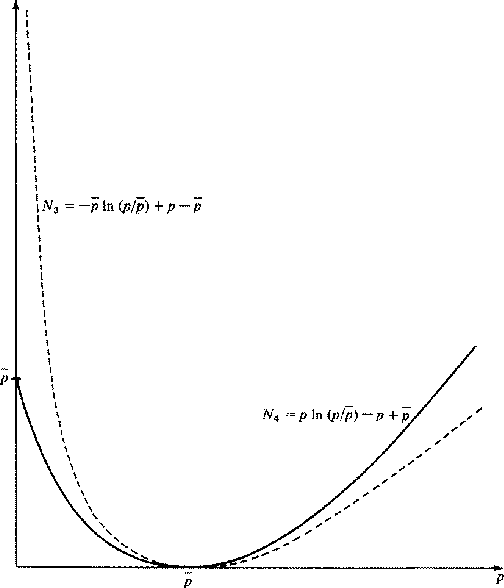Let us consider some inversion procedures which are coordinate-system invariant. We will restrict ourselves to physical problems in which we can identify a positive density function p as energy or dissipation per unit volume. Let us denote bythe value of this power as a function of space in the default model of the earth. The default model is the one we want to find when we have no measurements. It will often be one in which the material properties are constant functions of space. Now we will need some functions which we will call model norms. They have the properties of being positive for all (positive) p andand being minimized at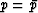.Some examples plotted in Figure 1 are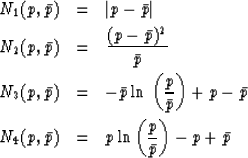Now let the adjustable earth properties be denoted by x, a function of space. We can choose x to minimize some volume integral of one of the model norms subject to the constraint that the model produce the required observations. Sometimes we have observations from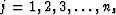source locations. We then need to compute the default power distribution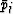for each. Then we can minimize a sum of volume integrals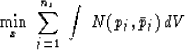subject to fitting all the data values.

It will be noted that the model-norm functions are all homogeneous of order 1. This means that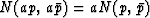for a > 0. This is our assurance that N is a volume density. Without this property we would have the difficulty that a sum of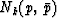over a set of subvolumes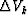would change as the mesh were refined. Coordinate-system invariance is provided by the usual rules for conversion of volume integrals from one coordinate system to another.

Now let us take up an example from filter theory which turns out to be related to maximum-entropy spectral estimation. We are given a known input spectrum R(Z) and are to find the finite length filter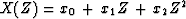whose output is as white as possible in the sense of minimizing the integral of N3 across the spectrum. Let the spectrum of the filter be.We have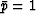and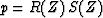.Thus, the minimization is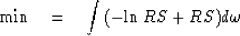Setting the derivative with respect to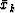equal to zero we have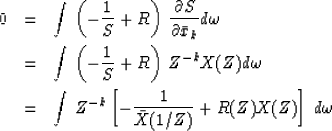Since we know that minimum-phase functions can represent any spectrum, we take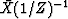to be expandable as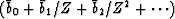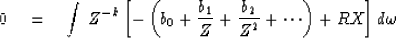We recall that this integral selects the coefficient of Z0 of the argument. If we suppose that the filter is constrained to have xk = 0 for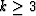,we get the familiar Toeplitz system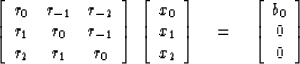Next: ROBUST MODELING Up: Data modeling by least Previous: HOUSEHOLDER TRANSFORMATIONS AND GOLUB'S
Stanford Exploration Project
10/30/1997